# A Novel Global Feature-Oriented Relational Triple Extraction Model based on Table Filling

## GRTE

### Table Filling Strategy

$$L = \set{\text{“N/A”, “MMH”, “MMT”, “MSH”, “MST”, “SMH”, “SMT”, “SS”}}$$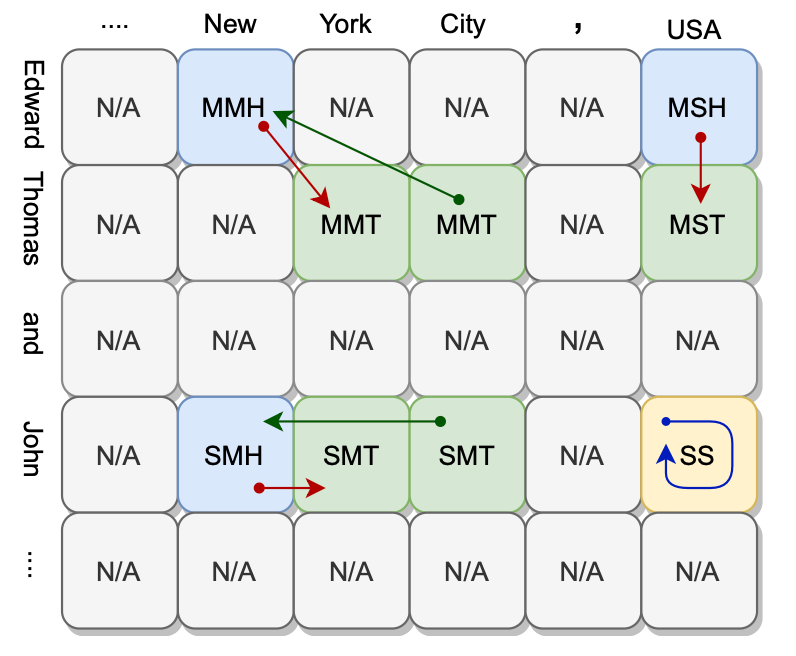EdwardNewMMHThomasCityMMT(Edward Thomas, New York City)M - M
EdwardNewMMHThomasYorkMMT(Edward Thomas, New York)M - M
EdwardUSAMSHThomasUSAMST(Edward Thomas, USA)M - S
JohnNewSMHJohnCitySMT(John, New York City)S - M
JohnNewSMHJohnYorkSMT(John, New York)S - M
JohnUSASS---(John, USA)S - S

### Model Details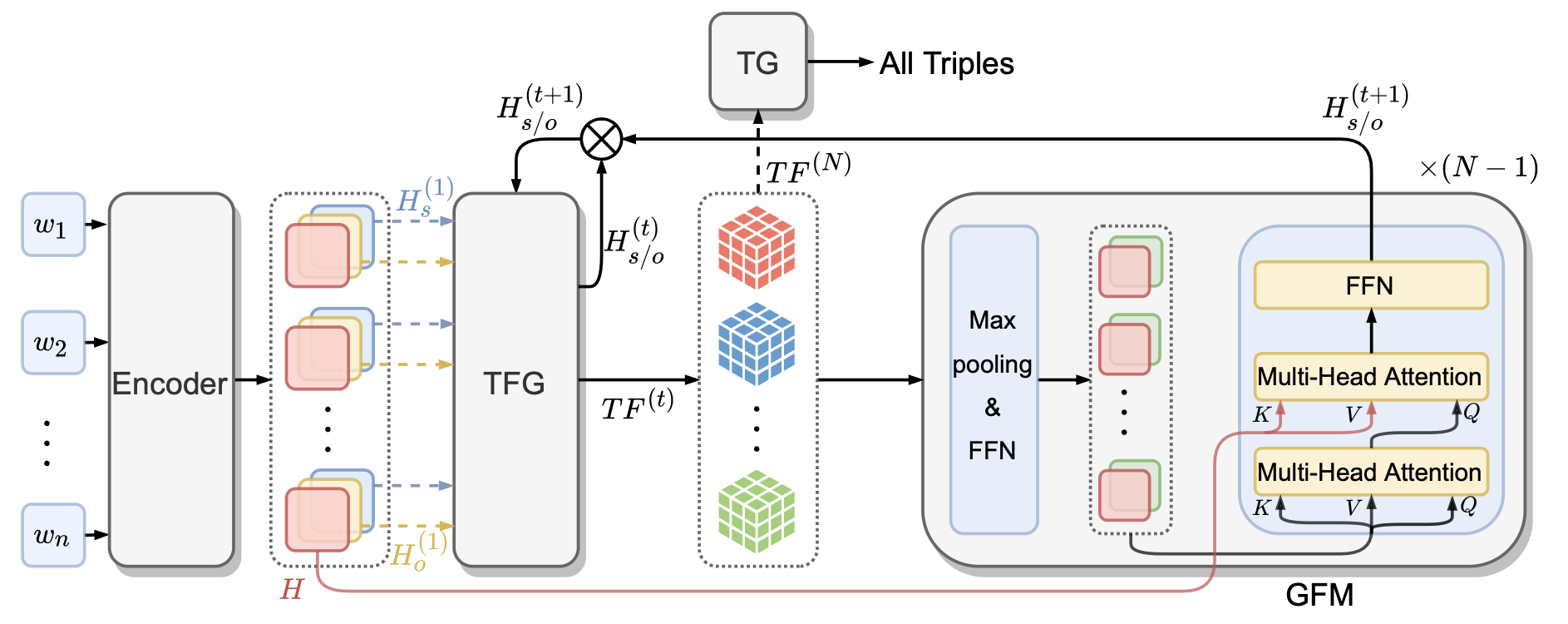#### Encoder Module

BERT作为预训练模型, 获得句子的表示$H \in \mathbb{R}^{n \times d_h}$, 然后用两个独立的FFN生成初始的Subject的特征$H_s^{(1)}$和Object的特征$H_o^{(1)}$:
\begin{aligned} &H_{s}^{(1)}=W_{1} H+b_{1} \\ &H_{o}^{(1)}=W_{2} H+b_{2} \end{aligned}

#### Table Feature Generation Module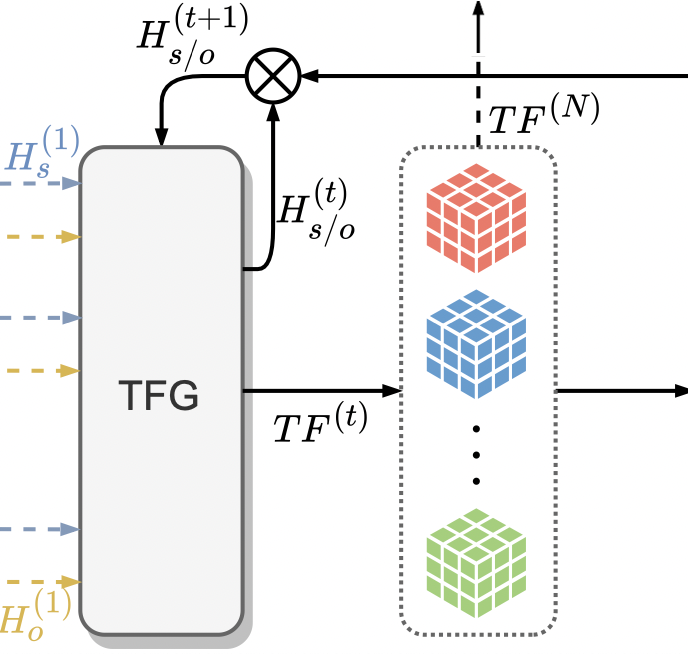TFG Module的输入$H_s, H_j$ 来自于TFG和GFM之间的迭代过程, 故记第$t$ 轮的SubjectObject的特征分别为$H_s^{(t)}, H_j^{(t)}$.

TFG会根据每种关系$r$, 利用Subject和Object特征分别生成一张Token Pair$(w_i, w_j)$ 之间的二维表格特征$TF_r^{(t)}$.

$$T F_{r}^{(t)}(i, j)=W_{r} \operatorname{ReLU}\left(H_{s, i}^{(t)} \circ H_{o, j}^{(t)}\right)+b_{r}$$

$TF^{(t)}_r \in \mathbb{R}^{n \times n \times |L|}, TF^{(t)} \in \mathbb{R}^{n \times n \times (|L| \times |R|)}$, 而且$TF_r^{(t)}$ 与$table_r$ 是同型的.

#### Global Feature Mining Module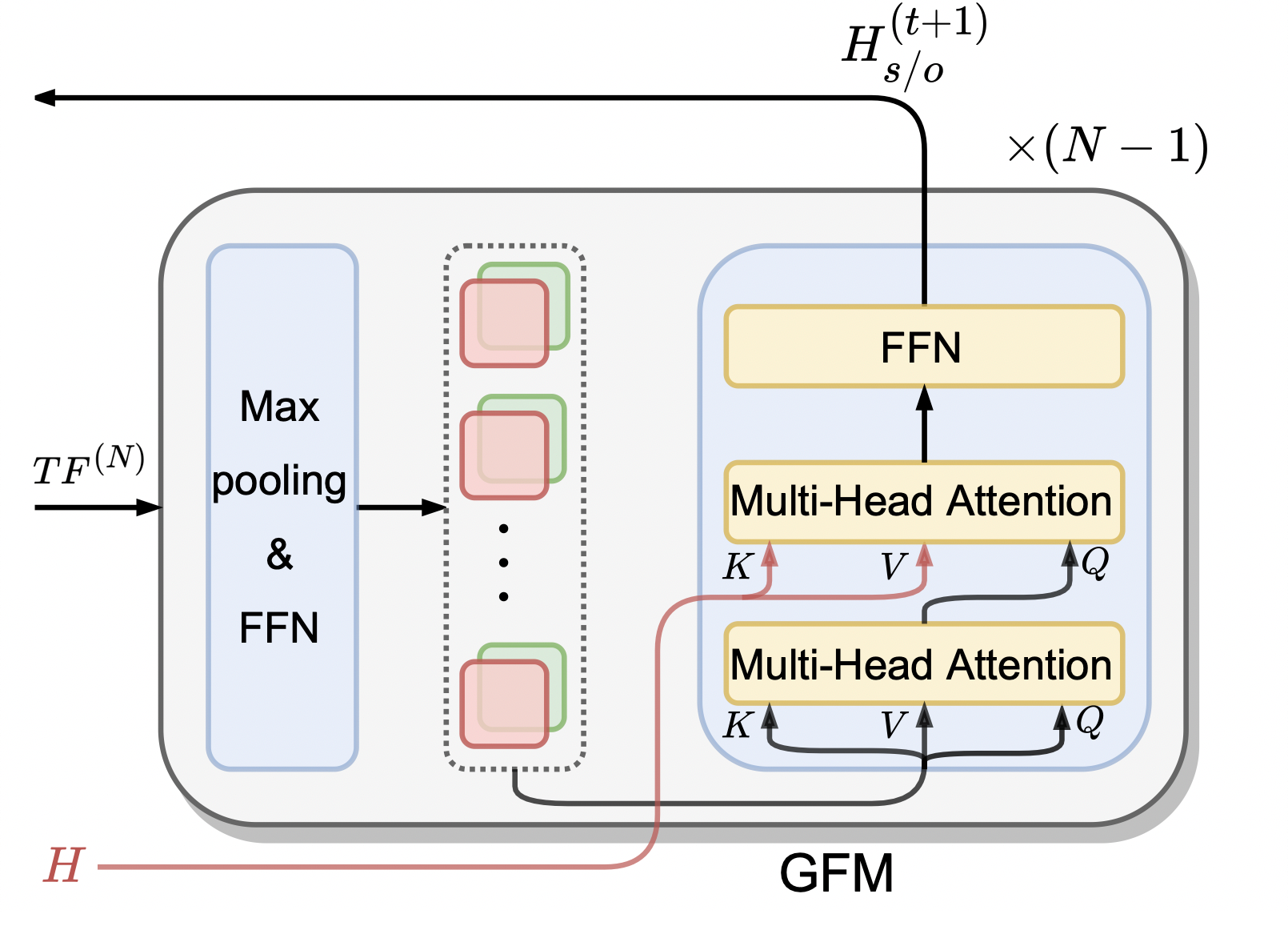GFM Module目的是同时考虑所有关系, 挖掘表格全局特征, 分三步走.

Step 1, 整合表格特征.

\begin{aligned} &T F_{s}^{(t)}=W_{s} \underset{s}{\operatorname{maxpool}}\left(T F^{(t)}\right)+b_{s} \\ &T F_{o}^{(t)}=W_{o} \underset{o}{\operatorname{maxpool}}\left(T F^{(t)}\right)+b_{o} \end{aligned}

$TF^{(t)} \in \mathbb{R}^{n \times n \times (|L|\times |R|)}, \underset{s/o}{\operatorname{maxpool}}(TF^{(t)}) \in \mathbb{R}^{n \times (|L| \times |R|)}$, 所以经过线性层$W_{s/o}$ 后维度为$TF_{s/o}^{(t)} \in \mathbb{R}^{n \times d_h}$, 大小又回到了$H$ 的大小.

Step 2, 挖掘全局特征.

\begin{aligned} \hat{T F}_{s / o}^{(t)} &=\text {MultiHeadSelfAtt}\left(T F_{s / o}^{(t)}\right) \\ \hat{H}_{(s / o)}^{(t+1)} &=\operatorname{MultiHeadAtt}\left(\hat{T F}_{s / o}^{(t)}, H, H\right) \\ H_{(s / o)}^{(t+1)} &=\operatorname{ReLU}\left(\hat{H}_{(s / o)}^{(t+1)} W+b\right) \end{aligned}

Step 3, 整合Subject / Object特征.

$$H_{(s / o)}^{(t+1)}=\operatorname{LayerNorm}\left(H_{(s / o)}^{(t)}+H_{(s / o)}^{(t+1)}\right)$$

GFM Module的最终输出$H_{(s / o)}^{(t+1)}$ 将会被重新送入TFG Module生成新的表特征$TF^{(t+1)}$, 进入下一轮迭代.

#### Triple Generation Module

TG Module的任务就是结合我们前面提到的Table Filling Strategy, 来解码出句子中所有的三元组.

\begin{aligned} \hat{\operatorname{table}}_{r}(i, j) &=\operatorname{softmax}\left(T F_{r}^{(N)}(i, j)\right) \\ \operatorname{table}_{r}(i, j) &=\underset{l \in L}{\operatorname{argmax}}\left(\hat{\operatorname{table}}_{r}(i, j)[l]\right) \end{aligned}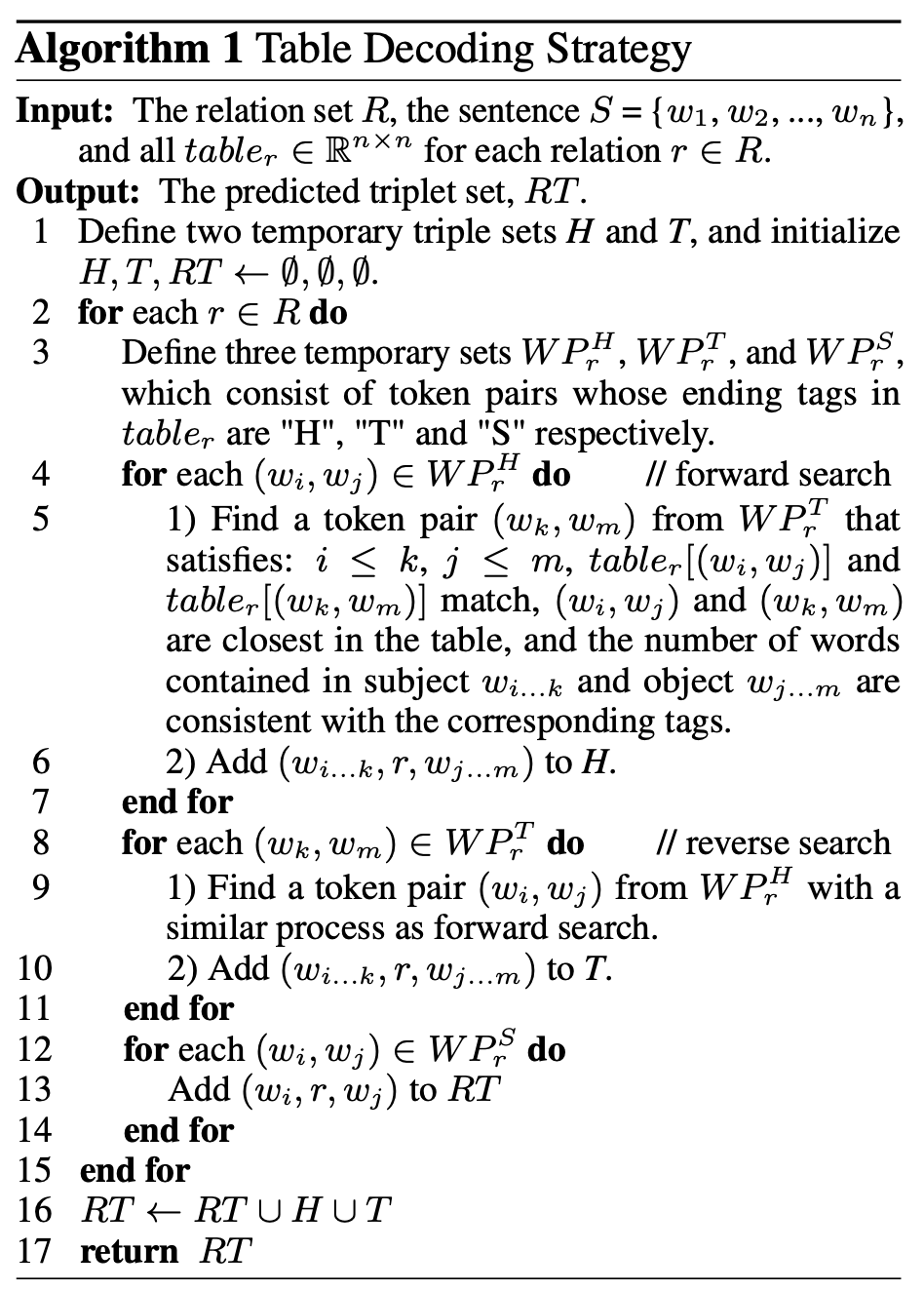• Forward Search: 找到离标签尾为H的格子最近的标签尾为T前缀与前者相同的格子, 解码作为一个三元组, 然后找下一个标签尾为H的格子.
• Reverse Search: 与前者相反, 找到离标签尾为T的格子最近的标签尾为H前缀与前者相同的格子, 解码作为一个三元组, 然后找下一个标签尾为T的格子. 与前者刚好互为补充.
• Single Token Pair: 找到标为SS的格子, 两个实体Token均为单个Token, 按行列直接解码出一个三元组, 然后找下一个SS格子.### Loss Function

\begin{aligned} \mathcal{L} &=\sum_{i=1}^{n} \sum_{j=1}^{n} \sum_{r=1}^{|R|}-\log p\left(y_{r,(i, j)}=t a b l e_{r}(i, j)\right) \\ &=\sum_{i=1}^{n} \sum_{j=1}^{n} \sum_{r=1}^{|R|}-\log t a \hat{b} l e_{r}(i, j)\left[y_{r,(i, j)}\right] \end{aligned}

## Experiments

### Datasets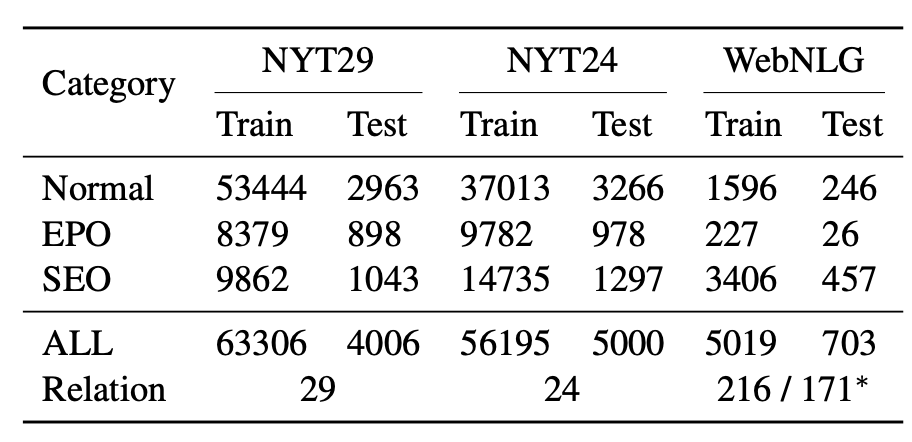NYT29的规模比NYT24要大一些.

### Experimental Results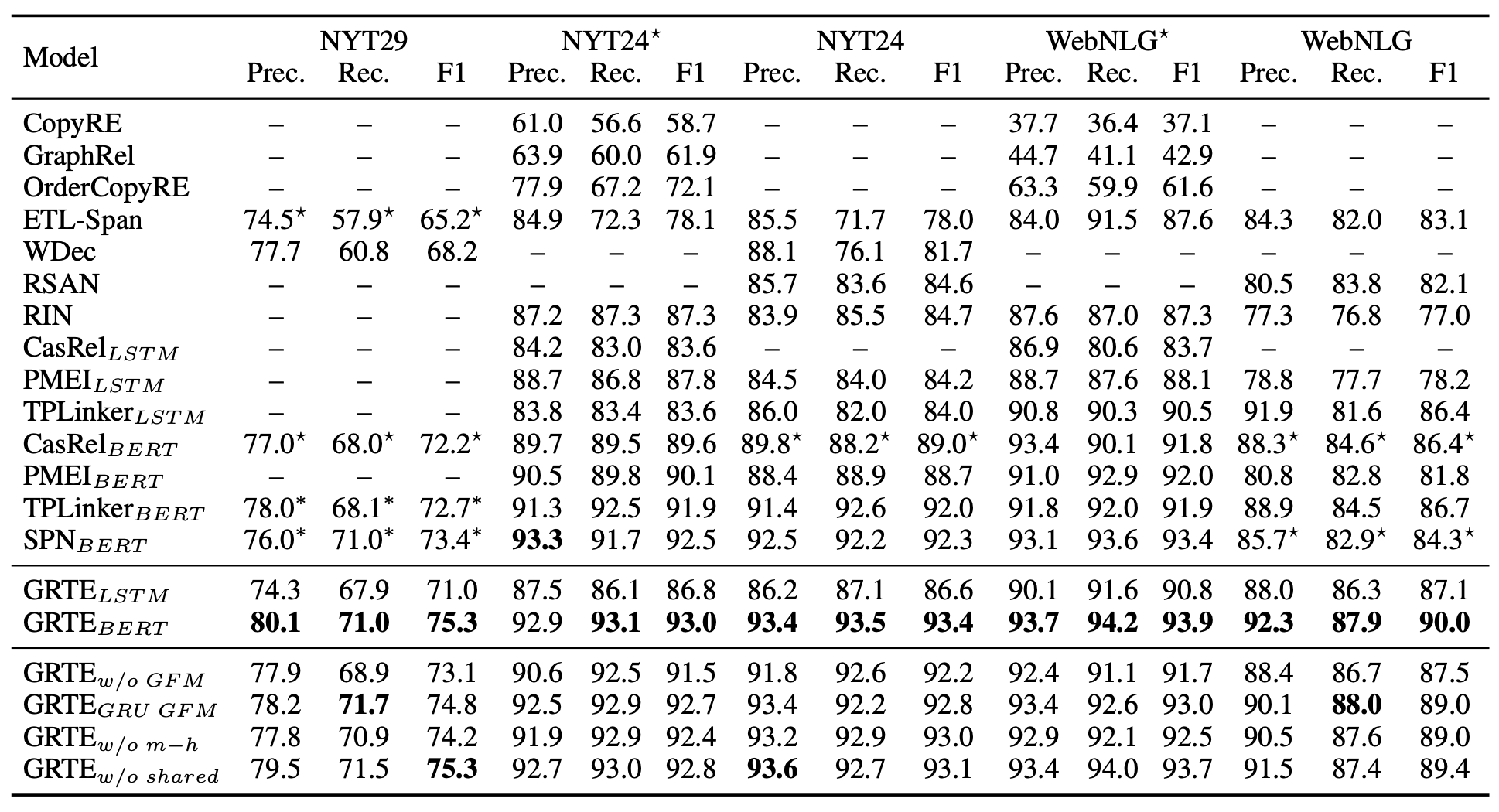• GRTE w/o GFM: 去掉GFM模块, 即去掉挖掘表格特征的迭代过程.
• GRTE GRU GIF: 用GRU代替GFM中的Transformer Decoder完成迭代过程.
• GRTE w/o m-h: 不用多头注意力而单头注意力.
• GRTE w/o shared: 在TFG和GFM中不共享参数, 即每轮迭代都使用不同的参数.

GRTE在不同数据集和不同迭代次数的F1曲线如下: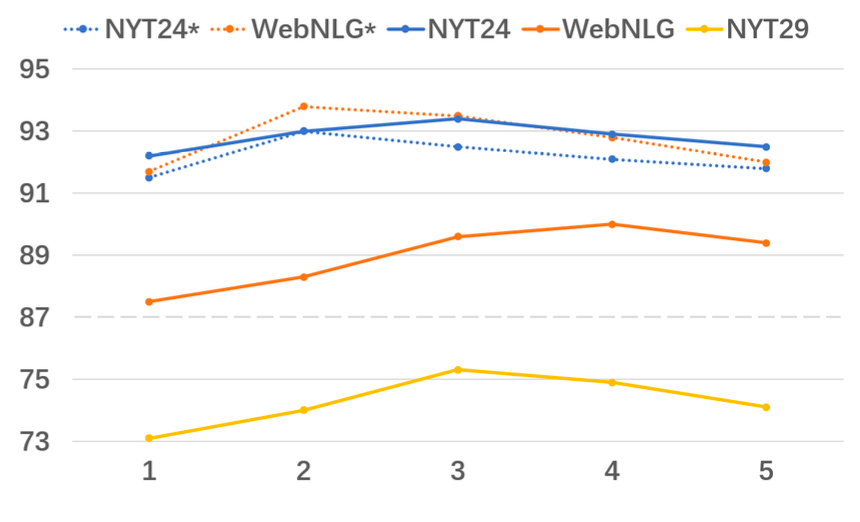### Analyses on Different Sentence Types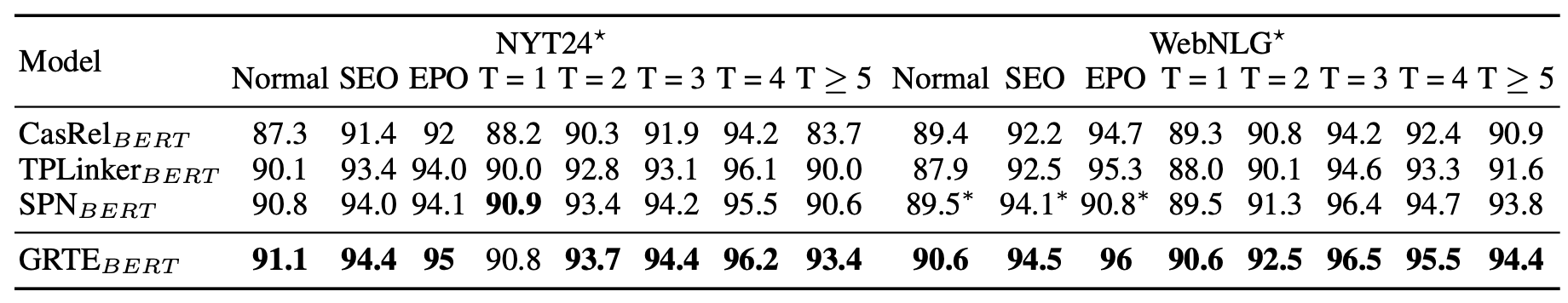### Analyses on Computational Efficiency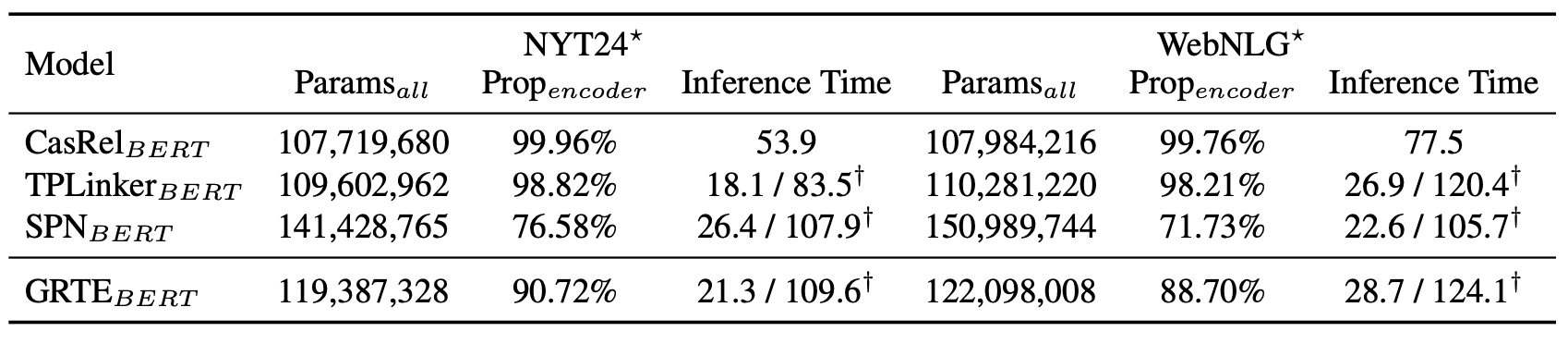## Summary

GRTE从三元组之间的相关性出发, 填补了前人忽视填表式方法中全局信息的空缺. 该方法基于全局特征, 通过独特的反复迭代方式不断提炼, 并从Relation的全局关联和Token Pair的全局关联两个方面挖掘表格中的全局特征, 也就是Generate - Mine - Integrate 过程.

上一篇SPN: Joint Entity and Relation Extraction with Set Prediction Networks
Joint Entity and Relation Extraction with Set Prediction Networks本文是论文Joint Entity and Relation Extraction with Set Pred
2022-06-22PRGC: Potential Relation and Global Correspondence Based Joint Relational Triple Extraction

2022-05-30
目录